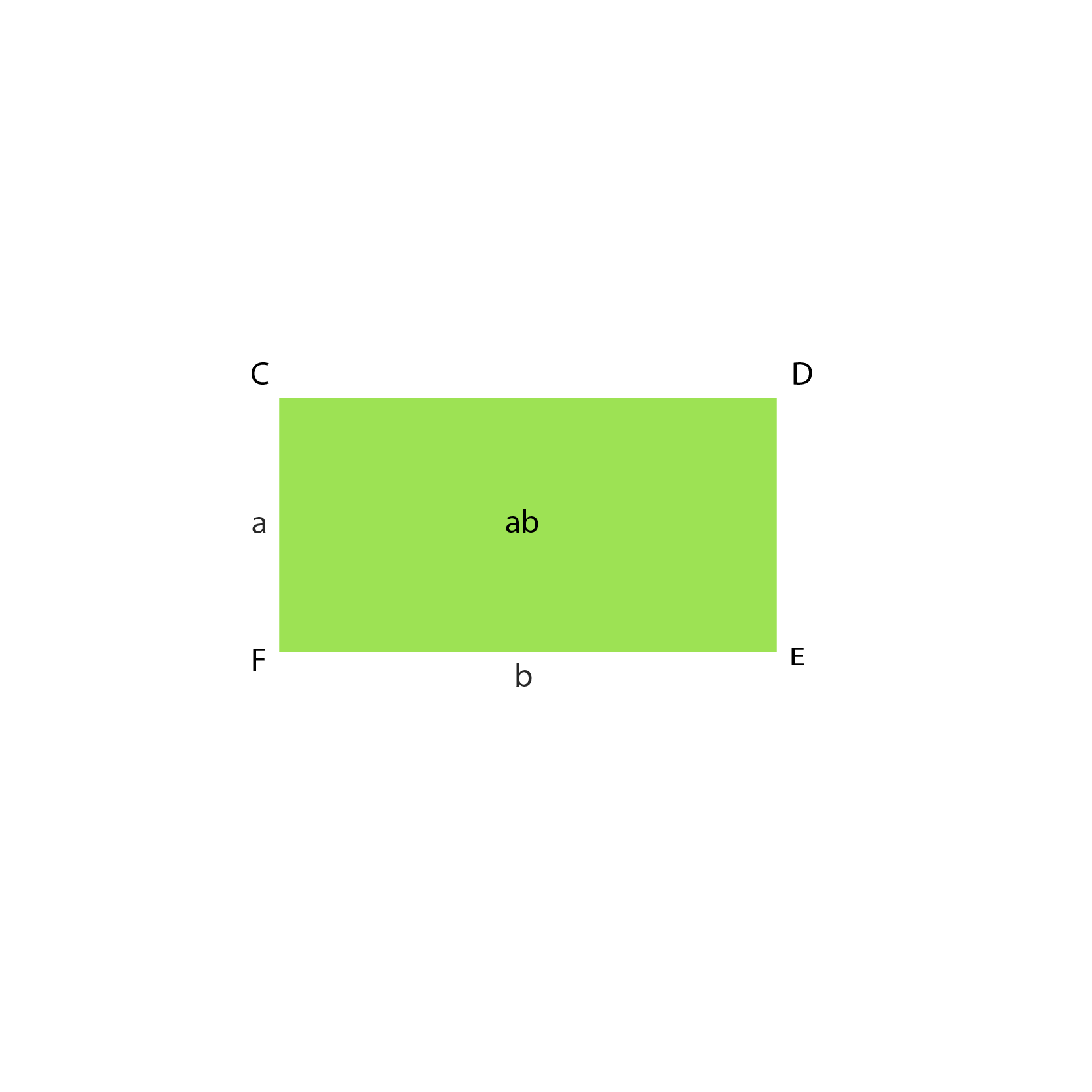Algebraic Identity (a - b)²

## Objective

To verify the algebraic identity (a - b)²= a² - 2ab + b²

### Algebraic Identity

• An algebraic identity is an equality, which is true for all values of the variables in the equality.
• The algebraic equations which are valid for all values of variables in them are called algebraic identities.
• In this way, algebraic identities are used in the computation of algebraic expressions and solving different polynomials
• Algebraic identities are also used for the factorization of polynomials.

### Pre-requisite Knowledge

SquareA square is a regular quadrilateral, which means that it has four equal sides and four equal angles of 90 degrees each.

Area of a Square

The product of the length of each side itself.

Formula: Area = side²

RectangleRectangle is a quadrilateral with four right angles and opposite sides are equal. The length of a rectangle is the size of the longer side, whereas the breadth is the size of the shorter side.

Area of a Rectangle

The product of its length and breadth

Formula: Area = length x breadth